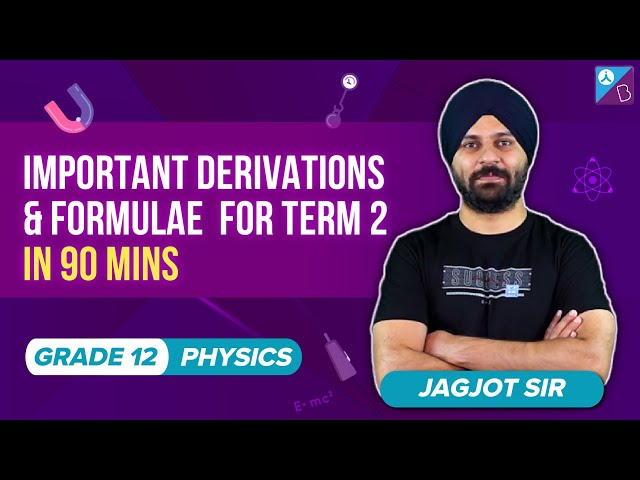Checkout JEE MAINS 2022 Question Paper Analysis : Checkout JEE MAINS 2022 Question Paper Analysis :

# Important Derivations for CBSE Class 12 Physics

Physics can be a scary ordeal for some and a very interesting subject for the rest. There are many scholars who want a program or some tips about important topics that support and help them in studying physics. With a proper preparation strategy, an idea about the important questions, derivations, and formulas in physics, a student can definitely score well in the main physics exam.

Here we would like to provide the important derivations of CBSE class 12 physics class 12 to help you score well in boards. Check the table below to learn some important derivations for Class 12 Physics.

List of Physics Derivations

The syllabus of CBSE class 12 physics is vast. It contains a total of 10 units or 15 chapters. It is important for students to know the important topics and derivations in class 12 physics to study efficiently. Given below are the important derivations for class 12 physics.
 Electrostatics(Unit  1) Coulomb’s Law in the form of vectors – $$\begin{array}{l}\vec{F_{12}}=-\vec{F_{21}}\end{array}$$ Application of Gauss theorem in the calculation of electric field Electric field intensity due to a thin infinite plane sheet of charge Electric field intensity of an electric dipole on equatorial line – $$\begin{array}{l}E_{R}=\frac{1}{4\pi \varepsilon _{0}}\frac{P}{r^{3}}\end{array}$$ Electric field intensity of an electric dipole on axial line – $$\begin{array}{l}E=\frac{1}{4\pi \varepsilon _{0}}\frac{2P}{r^{2}}\end{array}$$ Electric field intensity due to a uniformly charged spherical shell at various points The capacitance of a parallel plate capacitor with a dielectric medium The capacitance of a parallel plate capacitor without dielectric medium Electric potential due to a point charge – $$\begin{array}{l}V(r)=\frac{Q}{4\pi \varepsilon _{0}r}\end{array}$$
 Current Electricity(Unit 2) Application of Gauss theorem in the calculation of electric field intensity due to thin infinite plane sheet of charge uniformly charged spherical sphere The relation between current and drift velocity An expression for drift velocity Series and parallel connection of resistors
 Magnetic Effect of Current and Magnetism(Unit 3) Torque experienced by a current loop in a uniform magnetic field Ampere’s law and its application to an infinitely long straight wire. Straight and toroidal solenoids. The magnetic dipole moment of a revolving electron Apply Biot-Savart law to derive the expression for the magnetic field on the axis of a current-carrying circular loop. Cyclotrons The force between two parallel current-carrying conductors Torque on a magnetic dipole (bar magnet) in a uniform magnetic field. Current loop as a magnetic dipole and its magnetic dipole moment Force on a current-carrying conductor in a uniform magnetic field Force on a moving charge in uniform magnetic and electric field.
 Electromagnetic Induction And AC(Unit 4) Self and mutual induction Derivation of Emf induced in a rod moving in a uniform magnetic field. Impedance, reactance, and average power in series LCR, LR, LC, or CR circuit. The relation between peak and RMS value of the current Derivation for resonance in a series LCR Circuit
 Electromagnetic Waves(Unit 5) Derivation of displacement current
 Optics(Unit 6) Formula for Lens Refraction at convex surfaces The formula for Lens-Maker’s Diffraction using a single slit Derivation of the refractive index of minimum deviation in the prism Young Double Slit Experiment Derivation of the formula for angle of minimum deviation for the prism Polarisation
 Dual Nature of Radiation and Matter(Unit 7) Derivation of the De-Broglie Relation The relation between threshold frequency, frequency of the incident photon and cut-off potential
 Nuclei and Atoms(Unit 8) Derive $$\begin{array}{l}N=N_{0}e^{-\lambda t}\end{array}$$ Expression of the radius in hydrogen-like atoms
 Electronic Devices(Unit 9) Common emitter transistor amplifier Common base transistor amplifier
 Communication System(Unit 10) Frequency modulation and amplitude modulation

The above listed were a few important derivations of CBSE class 12 physics that are most likely to be asked in the coming board exams. While studying for the board exam, students can plan accordingly, giving priority to the above-mentioned topics.

## The video discusses the important derivations and formulae of Class 12 Physics.You can also download the important derivations in physics class 12 CBSE PDF to get well acquainted with all the derivations and answer related questions accurately in the class 12 board exams.

1. omesh shukla

can you please also leave a pdf for derivations?

2. Helena Haobam

Thanks a lot to byju app as it helps a lot since I m without the knowledge of any derivations

3. Sandeep

Nice this is very helpful for us
Thank you ☺😊🙏👍👌

4. this website and also the app both are making me confident in my subjects ,thank you byju’s .your always my favourite part of learning .the materials which you provide for us is so good.

5. Dixit dagar

Thank you byjus app